# Lecture 8 String 1 Concept of strings 2

• Slides: 26Lecture 8 String 1. Concept of strings 2. String and pointers 3. Array of strings 4. String operations 5. String library © Oxford University Press 2014. All rights reserved.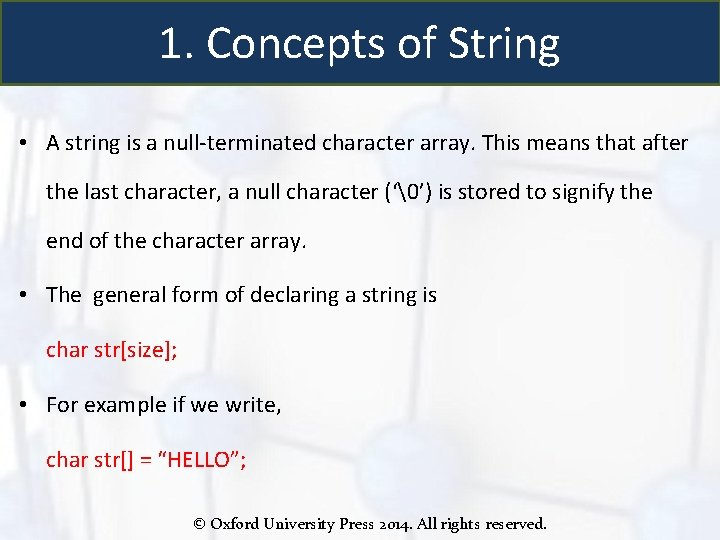1. Concepts of String • A string is a null-terminated character array. This means that after the last character, a null character (‘’) is stored to signify the end of the character array. • The general form of declaring a string is char str[size]; • For example if we write, char str[] = “HELLO”; © Oxford University Press 2014. All rights reserved.Concepts of String • We are declaring a character array with 5 characters namely, H, E, L, L and O. Besides, a null character (‘’) is stored at the end of the string. So, the internal representation of the string becomes HELLO‘’. • Note that to store a string of length 5, we need 5 + 1 locations (1 extra for the null character). • The name of the character array (or the string) is a pointer to the beginning of the string. © Oxford University Press 2014. All rights reserved.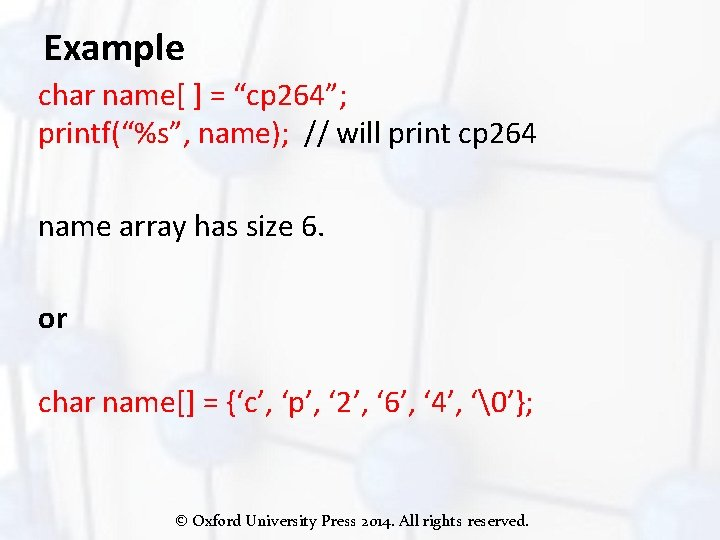Example char name[ ] = “cp 264”; printf(“%s”, name); // will print cp 264 name array has size 6. or char name[] = {‘c’, ‘p’, ‘ 2’, ‘ 6’, ‘ 4’, ‘’}; © Oxford University Press 2014. All rights reserved.Example use more space for string char name[ 20 ]; //name hold a string of 19 characters. name = ‘c’; // or name = 99; name = ‘p’; name = ‘ 2’; name = ‘ 6’; name = ‘ 4’; name = ‘’; // or name = 0; printf(“%s”, name); Alternative declaration initialization char name = “cp 264”; char name = {‘c’, ‘p’, ‘ 2’, ‘ 6’, ‘ 4’, ‘’}; © Oxford University Press 2014. All rights reserved.2. Pointers and Strings Since string is char array, array pointers and operations apply to string char name = “cp 264”; char *p; p = &name; printf("%cn", *p); // what this print? printf("%cn", *(p+3)); // what this print? printf("%sn", p+2); // what this print? © Oxford University Press 2014. All rights reserved.String pointer char *p 1 = "cp 264"; printf("%sn", p 1); printf("%cn", *(p 1+2)); Note: if a string is declared like char *a = “cp 264”; Then a can only be read. *(a+1) = ‘c’; is not allowed © Oxford University Press 2014. All rights reserved.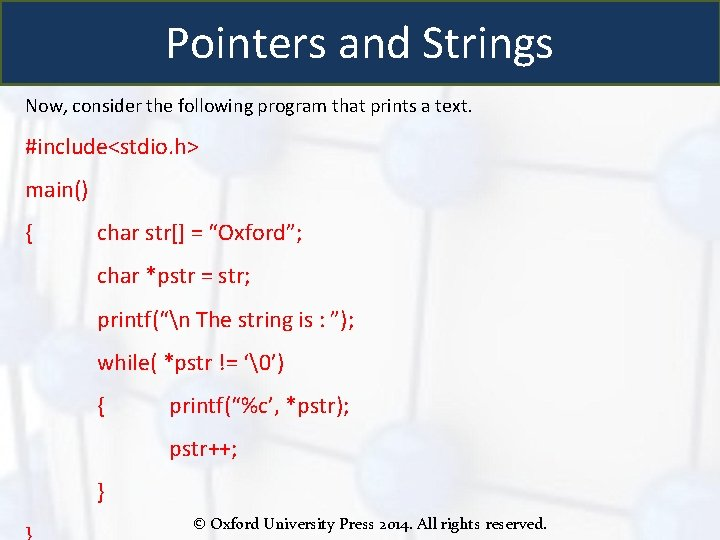Pointers and Strings Now, consider the following program that prints a text. #include<stdio. h> main() { char str[] = “Oxford”; char *pstr = str; printf(“n The string is : ”); while( *pstr != ‘’) { printf(“%c’, *pstr); pstr++; } © Oxford University Press 2014. All rights reserved.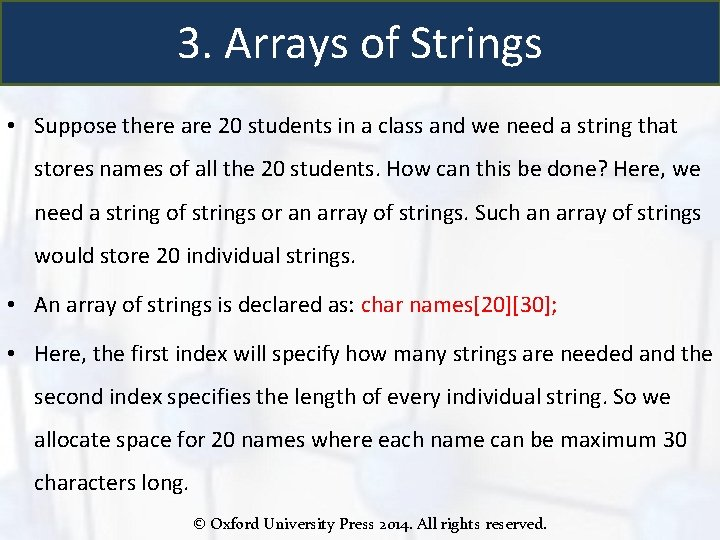3. Arrays of Strings • Suppose there are 20 students in a class and we need a string that stores names of all the 20 students. How can this be done? Here, we need a string of strings or an array of strings. Such an array of strings would store 20 individual strings. • An array of strings is declared as: char names; • Here, the first index will specify how many strings are needed and the second index specifies the length of every individual string. So we allocate space for 20 names where each name can be maximum 30 characters long. © Oxford University Press 2014. All rights reserved.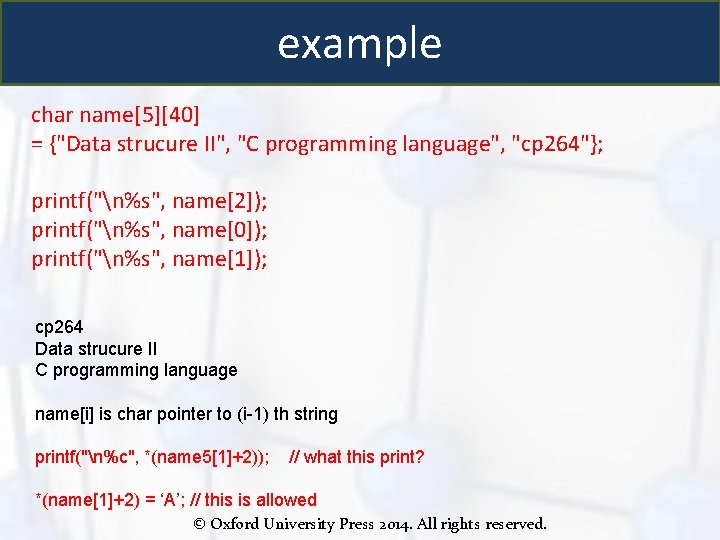example char name = {"Data strucure II", "C programming language", "cp 264"}; printf("n%s", name); printf("n%s", name); printf("n%s", name); cp 264 Data strucure II C programming language name[i] is char pointer to (i-1) th string printf("n%c", *(name 5+2)); // what this print? *(name+2) = ‘A’; // this is allowed © Oxford University Press 2014. All rights reserved.// array of char pointer, each pointer point to a string char *name[] = {"Data strucure II", "C programming language", "cp 264"}; printf("n%s", name); printf("n%s", name); printf("n%s", name); // *(name+2) = ‘A’; this is not allowed © Oxford University Press 2014. All rights reserved.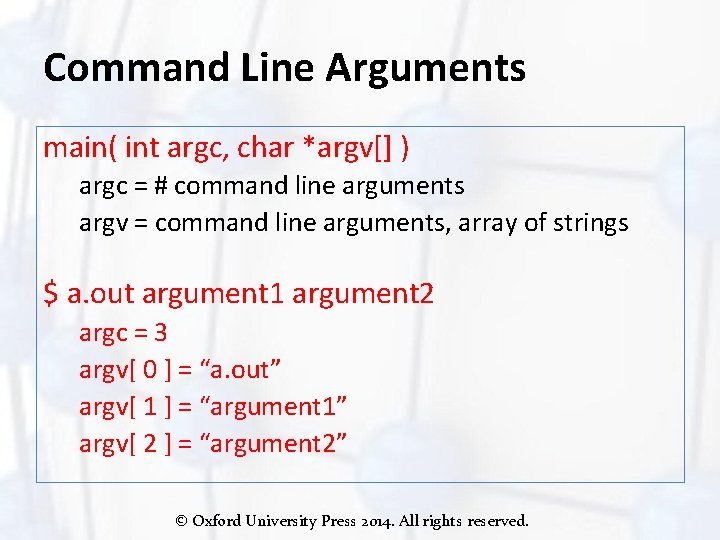Command Line Arguments main( int argc, char *argv[] ) argc = # command line arguments argv = command line arguments, array of strings \$ a. out argument 1 argument 2 argc = 3 argv[ 0 ] = “a. out” argv[ 1 ] = “argument 1” argv[ 2 ] = “argument 2” © Oxford University Press 2014. All rights reserved.4. String operations • • Copy string Read string Write string Length of a string Change case Concatenate/appending string Compare string … © Oxford University Press 2014. All rights reserved.String copy by pointer char a[] = “C programming language”; char c; int i; // copy string for (i = 0; *(a+i) !=''; i++) *(c+i) = *(a+i); *(c+i) = ‘’; // add NULL to end //print string for (i = 0; c[i] !=''; i++) printf("%c", c[i]); char d; char *p 1, *p 2; p 1 = a; p 2 = d; for (; *p 1!=''; p 1++, p 2++) *p 2 = *p 1; *p 2 = ''; // add NULL to end printf("%sn", d); © Oxford University Press 2014. All rights reserved.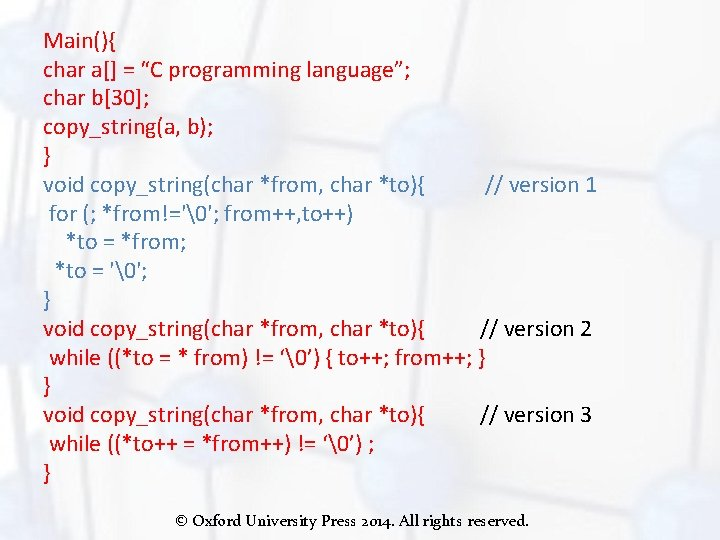Main(){ char a[] = “C programming language”; char b; copy_string(a, b); } void copy_string(char *from, char *to){ // version 1 for (; *from!=''; from++, to++) *to = *from; *to = ''; } void copy_string(char *from, char *to){ // version 2 while ((*to = * from) != ‘’) { to++; from++; } } void copy_string(char *from, char *to){ // version 3 while ((*to++ = *from++) != ‘’) ; } © Oxford University Press 2014. All rights reserved.Reading Strings If we declare a string by writing char str; Then str can be read from the user by using three ways using scanf function using gets() function using getchar() function repeatedly str can be read using scanf() by writing scanf(“%s”, str); str can be read by writing gets(str); gets() takes the starting address of the string which will hold the input. The string inputted using gets() is automatically terminated with a null character. © Oxford University Press 2014. All rights reserved.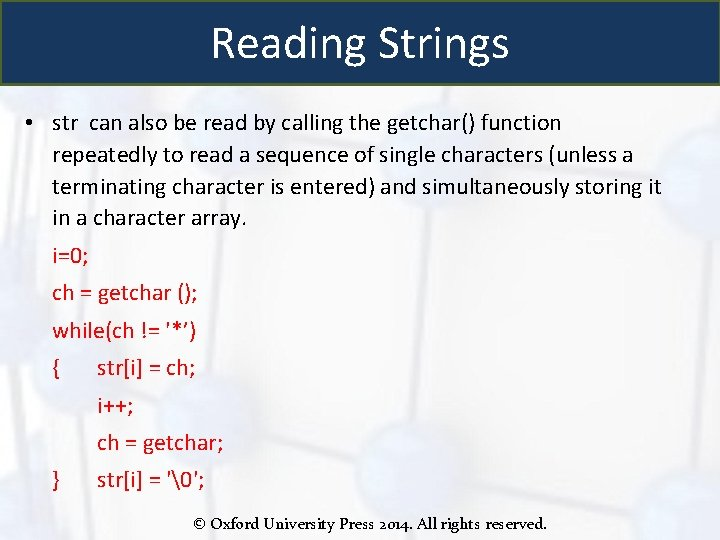Reading Strings • str can also be read by calling the getchar() function repeatedly to read a sequence of single characters (unless a terminating character is entered) and simultaneously storing it in a character array. i=0; ch = getchar (); while(ch != '*’) { str[i] = ch; i++; ch = getchar; } str[i] = ''; © Oxford University Press 2014. All rights reserved.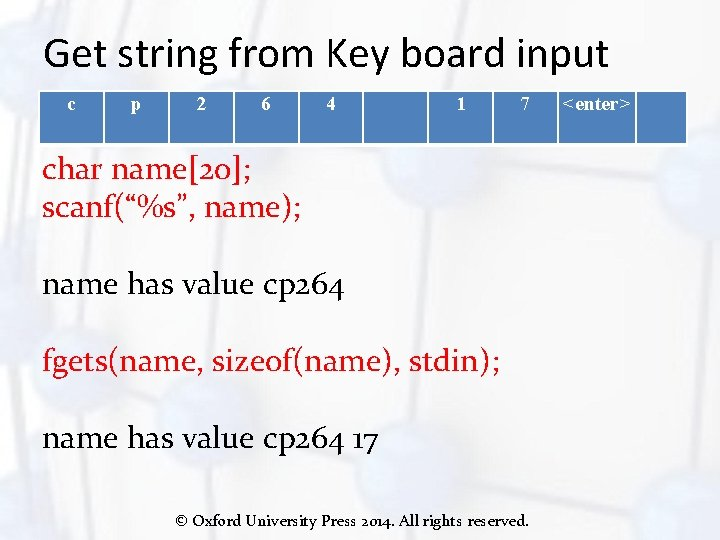Get string from Key board input c p 2 6 4 1 7 char name; scanf(“%s”, name); name has value cp 264 fgets(name, sizeof(name), stdin); name has value cp 264 17 © Oxford University Press 2014. All rights reserved. <enter>Writing Strings • Strings can be displayed on screen using three ways using printf() function using puts() function using putchar() function repeatedly • str can be displayed using printf() by writing printf(“%s”, str); • str can be displayed by writing puts(str); © Oxford University Press 2014. All rights reserved.Writing Strings str can also be written by calling the putchar() repeatedly to print a sequence of single characters i=0; while(str[i] != '’) { putchar(str[i]); i++; } © Oxford University Press 2014. All rights reserved.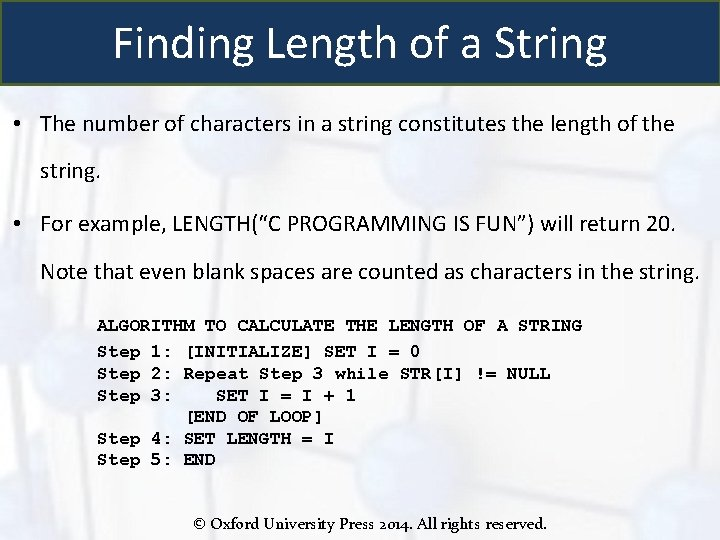Finding Length of a String • The number of characters in a string constitutes the length of the string. • For example, LENGTH(“C PROGRAMMING IS FUN”) will return 20. Note that even blank spaces are counted as characters in the string. ALGORITHM TO CALCULATE THE LENGTH OF A STRING Step 1: [INITIALIZE] SET I = 0 Step 2: Repeat Step 3 while STR[I] != NULL Step 3: SET I = I + 1 [END OF LOOP] Step 4: SET LENGTH = I Step 5: END © Oxford University Press 2014. All rights reserved.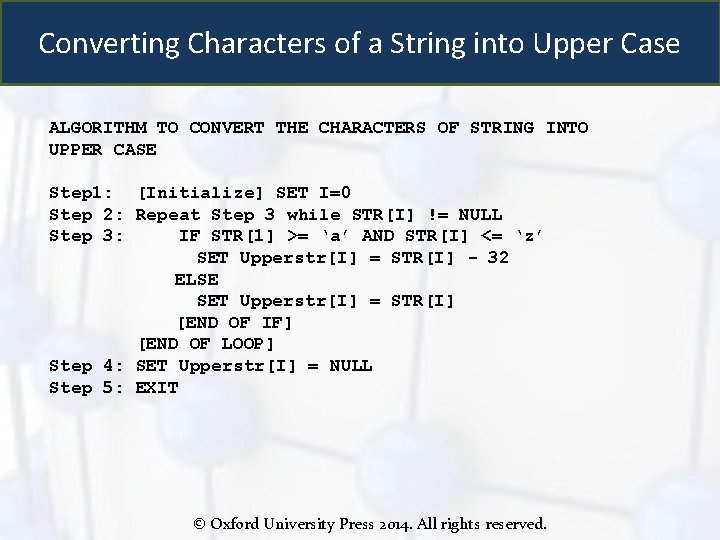Converting Characters of a String into Upper Case ALGORITHM TO CONVERT THE CHARACTERS OF STRING INTO UPPER CASE Step 1: [Initialize] SET I=0 Step 2: Repeat Step 3 while STR[I] != NULL Step 3: IF STR >= ‘a’ AND STR[I] <= ‘z’ SET Upperstr[I] = STR[I] - 32 ELSE SET Upperstr[I] = STR[I] [END OF IF] [END OF LOOP] Step 4: SET Upperstr[I] = NULL Step 5: EXIT © Oxford University Press 2014. All rights reserved.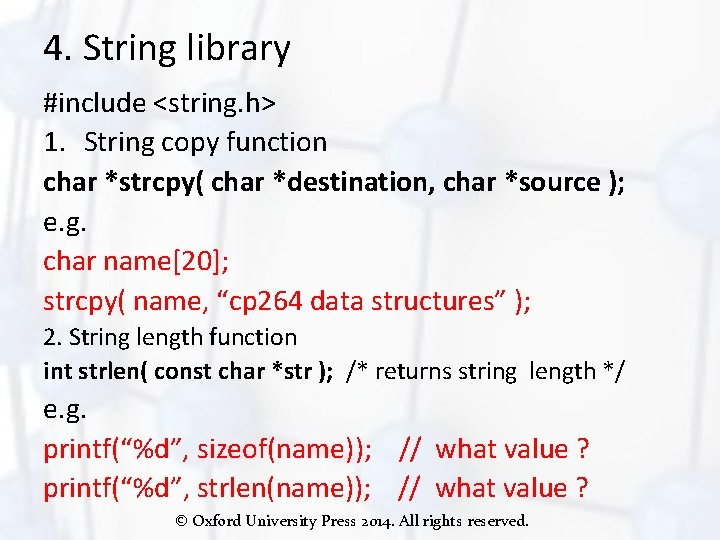4. String library #include <string. h> 1. String copy function char *strcpy( char *destination, char *source ); e. g. char name; strcpy( name, “cp 264 data structures” ); 2. String length function int strlen( const char *str ); /* returns string length */ e. g. printf(“%d”, sizeof(name)); // what value ? printf(“%d”, strlen(name)); // what value ? © Oxford University Press 2014. All rights reserved.3. Memory copy function void *memcpy(void *str 1, const void *str 2, size_t n); // copies n characters from memory area str 2 to memory area str 1. e. . g const char src = " cp 264 data structures "; char dest; memcpy(dest, src, strlen(src)+1); © Oxford University Press 2014. All rights reserved.4. String library #include <string. h> char *strcpy( char *destination, char *source ); e. g. char name; strcpy( name, “cp 264 data structures” ); const char src = "memory copy function"; char dest; printf("Before memcpy dest = %sn", dest); memcpy(dest, src, strlen(src)+1); • Get string lenghth int strlen( const char *str ); /* returns string length */ e. g. printf(“%d”, sizeof(name)); // what value ? printf(“%d”, strlen(name)); // what value ? © Oxford University Press 2014. All rights reserved.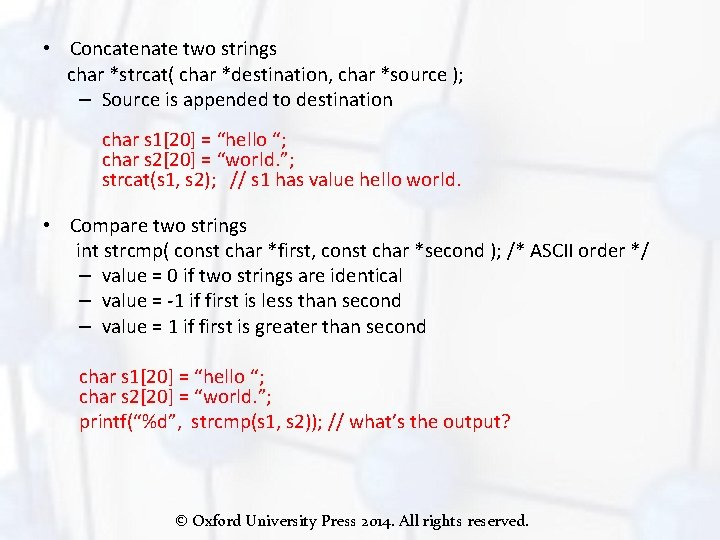• Concatenate two strings char *strcat( char *destination, char *source ); – Source is appended to destination char s 1 = “hello “; char s 2 = “world. ”; strcat(s 1, s 2); // s 1 has value hello world. • Compare two strings int strcmp( const char *first, const char *second ); /* ASCII order */ – value = 0 if two strings are identical – value = -1 if first is less than second – value = 1 if first is greater than second char s 1 = “hello “; char s 2 = “world. ”; printf(“%d”, strcmp(s 1, s 2)); // what’s the output? © Oxford University Press 2014. All rights reserved.